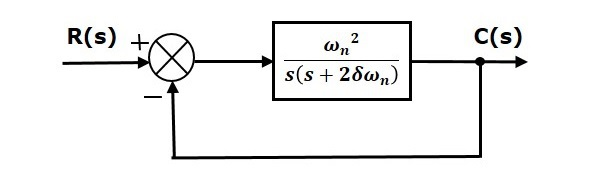# Response of Second Order System

In this chapter, let us discuss the time response of second order system. Consider the following block diagram of closed loop control system. Here, an open loop transfer function, $\frac{\omega ^2_n}{s(s+2\delta \omega_n)}$ is connected with a unity negative feedback.We know that the transfer function of the closed loop control system having unity negative feedback as

$$\frac{C(s)}{R(s)}=\frac{G(s)}{1+G(s)}$$

Substitute, $G(s)=\frac{\omega ^2_n}{s(s+2\delta \omega_n)}$ in the above equation.

$$\frac{C(s)}{R(s)}=\frac{\left (\frac{\omega ^2_n}{s(s+2\delta \omega_n)} \right )}{1+ \left ( \frac{\omega ^2_n}{s(s+2\delta \omega_n)} \right )}=\frac{\omega _n^2}{s^2+2\delta \omega _ns+\omega _n^2}$$

The power of ‘s’ is two in the denominator term. Hence, the above transfer function is of the second order and the system is said to be the second order system.

The characteristic equation is -

$$s^2+2\delta \omega _ns+\omega _n^2=0$$

The roots of characteristic equation are -

$$s=\frac{-2\omega \delta _n\pm \sqrt{(2\delta\omega _n)^2-4\omega _n^2}}{2}=\frac{-2(\delta\omega _n\pm \omega _n\sqrt{\delta ^2-1})}{2}$$

$$\Rightarrow s=-\delta \omega_n \pm \omega _n\sqrt{\delta ^2-1}$$

• The two roots are imaginary when δ = 0.
• The two roots are real and equal when δ = 1.
• The two roots are real but not equal when δ > 1.
• The two roots are complex conjugate when 0 < δ < 1.

We can write $C(s)$ equation as,

$$C(s)=\left ( \frac{\omega _n^2}{s^2+2\delta\omega_ns+\omega_n^2} \right )R(s)$$

Where,

• C(s) is the Laplace transform of the output signal, c(t)

• R(s) is the Laplace transform of the input signal, r(t)

• ωn is the natural frequency

• δ is the damping ratio.

Follow these steps to get the response (output) of the second order system in the time domain.

• Take Laplace transform of the input signal, $r(t)$.

• Consider the equation, $C(s)=\left ( \frac{\omega _n^2}{s^2+2\delta\omega_ns+\omega_n^2} \right )R(s)$

• Substitute $R(s)$ value in the above equation.

• Do partial fractions of $C(s)$ if required.

• Apply inverse Laplace transform to $C(s)$.

## Step Response of Second Order System

Consider the unit step signal as an input to the second order system.

Laplace transform of the unit step signal is,

$$R(s)=\frac{1}{s}$$

We know the transfer function of the second order closed loop control system is,

$$\frac{C(s)}{R(s)}=\frac{\omega _n^2}{s^2+2\delta\omega_ns+\omega_n^2}$$

### Case 1: δ = 0

Substitute, $\delta = 0$ in the transfer function.

$$\frac{C(s)}{R(s)}=\frac{\omega_n^2}{s^2+\omega_n^2}$$

$$\Rightarrow C(s)=\left( \frac{\omega_n^2}{s^2+\omega_n^2} \right )R(s)$$

Substitute, $R(s) = \frac{1}{s}$ in the above equation.

$$C(s)=\left( \frac{\omega_n^2}{s^2+\omega_n^2} \right )\left( \frac{1}{s} \right )=\frac{\omega_n^2}{s(s^2+\omega_n^2)}$$

Apply inverse Laplace transform on both the sides.

$$c(t)=\left ( 1-\cos(\omega_n t) \right )u(t)$$

So, the unit step response of the second order system when $/delta = 0$ will be a continuous time signal with constant amplitude and frequency.

### Case 2: δ = 1

Substitute, $/delta = 1$ in the transfer function.

$$\frac{C(s)}{R(s)}=\frac{\omega_n^2}{s^2+2\omega_ns+\omega_n^2}$$

$$\Rightarrow C(s)=\left( \frac{\omega_n^2}{(s+\omega_n)^2} \right)R(s)$$

Substitute, $R(s) = \frac{1}{s}$ in the above equation.

$$C(s)=\left( \frac{\omega_n^2}{(s+\omega_n)^2} \right)\left ( \frac{1}{s} \right)=\frac{\omega_n^2}{s(s+\omega_n)^2}$$

Do partial fractions of $C(s)$.

$$C(s)=\frac{\omega_n^2}{s(s+\omega_n)^2}=\frac{A}{s}+\frac{B}{s+\omega_n}+\frac{C}{(s+\omega_n)^2}$$

After simplifying, you will get the values of A, B and C as $1,\: -1\: and \: −\omega _n$ respectively. Substitute these values in the above partial fraction expansion of $C(s)$.

$$C(s)=\frac{1}{s}-\frac{1}{s+\omega_n}-\frac{\omega_n}{(s+\omega_n)^2}$$

Apply inverse Laplace transform on both the sides.

$$c(t)=(1-e^{-\omega_nt}-\omega _nte^{-\omega_nt})u(t)$$

So, the unit step response of the second order system will try to reach the step input in steady state.

### Case 3: 0 < δ < 1

We can modify the denominator term of the transfer function as follows −

$$s^2+2\delta\omega_ns+\omega_n^2=\left \{ s^2+2(s)(\delta \omega_n)+(\delta \omega_n)^2 \right \}+\omega_n^2-(\delta\omega_n)^2$$

$$=(s+\delta\omega_n)^2+\omega_n^2(1-\delta^2)$$

The transfer function becomes,

$$\frac{C(s)}{R(s)}=\frac{\omega_n^2}{(s+\delta\omega_n)^2+\omega_n^2(1-\delta^2)}$$

$$\Rightarrow C(s)=\left( \frac{\omega_n^2}{(s+\delta\omega_n)^2+\omega_n^2(1-\delta^2)} \right )R(s)$$

Substitute, $R(s) = \frac{1}{s}$ in the above equation.

$$C(s)=\left( \frac{\omega_n^2}{(s+\delta\omega_n)^2+\omega_n^2(1-\delta^2)} \right )\left( \frac{1}{s} \right )=\frac{\omega_n^2}{s\left ((s+\delta\omega_n)^2+\omega_n^2(1-\delta^2) \right)}$$

Do partial fractions of $C(s)$.

$$C(s)=\frac{\omega_n^2}{s\left ((s+\delta\omega_n)^2+\omega_n^2(1-\delta^2) \right)}=\frac{A}{s}+\frac{Bs+C}{(s+\delta\omega_n)^2+\omega_n^2(1-\delta^2)}$$

After simplifying, you will get the values of A, B and C as $1,\: -1 \: and \: −2\delta \omega _n$ respectively. Substitute these values in the above partial fraction expansion of C(s).

$$C(s)=\frac{1}{s}-\frac{s+2\delta\omega_n}{(s+\delta\omega_n)^2+\omega_n^2(1-\delta^2)}$$

$$C(s)=\frac{1}{s}-\frac{s+\delta\omega_n}{(s+\delta\omega_n)^2+\omega_n^2(1-\delta^2)}-\frac{\delta\omega_n}{(s+\delta\omega_n)^2+\omega_n^2(1-\delta^2)}$$

$C(s)=\frac{1}{s}-\frac{(s+\delta\omega_n)}{(s+\delta\omega_n)^2+(\omega_n\sqrt{1-\delta^2})^2}-\frac{\delta}{\sqrt{1-\delta^2}}\left ( \frac{\omega_n\sqrt{1-\delta^2}}{(s+\delta\omega_n)^2+(\omega_n\sqrt{1-\delta^2})^2} \right )$

Substitute, $\omega_n\sqrt{1-\delta^2}$ as $\omega_d$ in the above equation.

$$C(s)=\frac{1}{s}-\frac{(s+\delta\omega_n)}{(s+\delta\omega_n)^2+\omega_d^2}-\frac{\delta}{\sqrt{1-\delta^2}}\left ( \frac{\omega_d}{(s+\delta\omega_n)^2+\omega_d^2} \right )$$

Apply inverse Laplace transform on both the sides.

$$c(t)=\left ( 1-e^{-\delta \omega_nt}\cos(\omega_dt)-\frac{\delta}{\sqrt{1-\delta^2}}e^{-\delta\omega_nt}\sin(\omega_dt) \right )u(t)$$

$$c(t)=\left ( 1-\frac{e^{-\delta\omega_nt}}{\sqrt{1-\delta^2}}\left ( (\sqrt{1-\delta^2})\cos(\omega_dt)+\delta \sin(\omega_dt) \right ) \right )u(t)$$

If $\sqrt{1-\delta^2}=\sin(\theta)$, then ‘δ’ will be cos(θ). Substitute these values in the above equation.

$$c(t)=\left ( 1-\frac{e^{-\delta\omega_nt}}{\sqrt{1-\delta^2}}(\sin(\theta)\cos(\omega_dt)+\cos(\theta)\sin(\omega_dt)) \right )u(t)$$

$$\Rightarrow c(t)=\left ( 1-\left ( \frac{e^{-\delta\omega_nt}}{\sqrt{1-\delta^2}} \right )\sin(\omega_dt+\theta) \right )u(t)$$

So, the unit step response of the second order system is having damped oscillations (decreasing amplitude) when ‘δ’ lies between zero and one.

### Case 4: δ > 1

We can modify the denominator term of the transfer function as follows −

$$s^2+2\delta\omega_ns+\omega_n^2=\left \{ s^2+2(s)(\delta\omega_n)+(\delta\omega_n)^2 \right \}+\omega_n^2-(\delta\omega_n)^2$$

$$=\left ( s+\delta\omega_n \right )^2-\omega_n^2\left ( \delta^2-1 \right )$$

The transfer function becomes,

$$\frac{C(s)}{R(s)}=\frac{\omega_n^2}{(s+\delta\omega_n)^2-\omega_n^2(\delta^2-1)}$$

$$\Rightarrow C(s)=\left ( \frac{\omega_n^2}{(s+\delta\omega_n)^2-\omega_n^2(\delta^2-1)} \right )R(s)$$

Substitute, $R(s) = \frac{1}{s}$ in the above equation.

$C(s)=\left ( \frac{\omega_n^2}{(s+\delta\omega_n)^2-(\omega_n\sqrt{\delta^2-1})^2} \right )\left ( \frac{1}{s} \right )=\frac{\omega_n^2}{s(s+\delta\omega_n+\omega_n\sqrt{\delta^2-1})(s+\delta\omega_n-\omega_n\sqrt{\delta^2-1})}$

Do partial fractions of $C(s)$.

$$C(s)=\frac{\omega_n^2}{s(s+\delta\omega_n+\omega_n\sqrt{\delta^2-1})(s+\delta\omega_n-\omega_n\sqrt{\delta^2-1})}$$

$$=\frac{A}{s}+\frac{B}{s+\delta\omega_n+\omega_n\sqrt{\delta^2-1}}+\frac{C}{s+\delta\omega_n-\omega_n\sqrt{\delta^2-1}}$$

After simplifying, you will get the values of A, B and C as 1, $\frac{1}{2(\delta+\sqrt{\delta^2-1})(\sqrt{\delta^2-1})}$ and $\frac{-1}{2(\delta-\sqrt{\delta^2-1})(\sqrt{\delta^2-1})}$ respectively. Substitute these values in above partial fraction expansion of $C(s)$.

$$C(s)=\frac{1}{s}+\frac{1}{2(\delta+\sqrt{\delta^2-1})(\sqrt{\delta^2-1})}\left ( \frac{1}{s+\delta\omega_n+\omega_n\sqrt{\delta^2-1}} \right )-\left ( \frac{1}{2(\delta-\sqrt{\delta^2-1})(\sqrt{\delta^2-1})} \right )\left ( \frac{1}{s+\delta\omega_n-\omega_n\sqrt{\delta^2-1}} \right )$$

Apply inverse Laplace transform on both the sides.

$c(t)=\left ( 1+\left ( \frac{1}{2(\delta+\sqrt{\delta^2-1})(\sqrt{\delta^2-1})} \right )e^{-(\delta\omega_n+\omega_n\sqrt{\delta^2-1})t}-\left ( \frac{1}{2(\delta-\sqrt{\delta^2-1})(\sqrt{\delta^2-1})} \right )e^{-(\delta\omega_n-\omega_n\sqrt{\delta^2-1})t} \right )u(t)$

Since it is over damped, the unit step response of the second order system when δ > 1 will never reach step input in the steady state.

## Impulse Response of Second Order System

The impulse response of the second order system can be obtained by using any one of these two methods.

• Follow the procedure involved while deriving step response by considering the value of $R(s)$ as 1 instead of $\frac{1}{s}$.

• Do the differentiation of the step response.

The following table shows the impulse response of the second order system for 4 cases of the damping ratio.

Condition of Damping ratio Impulse response for t ≥ 0

δ = 0

$\omega_n\sin(\omega_nt)$

δ = 1

$\omega_n^2te^{-\omega_nt}$

0 < δ < 1

$\left ( \frac{\omega_ne^{-\delta\omega_nt}}{\sqrt{1-\delta^2}} \right )\sin(\omega_dt)$

δ > 1

$\left ( \frac{\omega_n}{2\sqrt{\delta^2-1}} \right )\left ( e^{-(\delta\omega_n-\omega_n\sqrt{\delta^2-1})t}-e^{-(\delta\omega_n+\omega_n\sqrt{\delta^2-1})t} \right )$